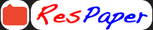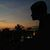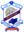Trending ▼   ResFinder# ICSE Class X Prelims 2019 : Bengali (Don Bosco School, Liluah, Howrah)

8 pages, 9 questions, 0 questions with responses, 0 total responses,00Pritam Sarkar Don Bosco School, Liluah, Howrah
+Fave Message
 Home > pritam003 >   F Also featured on: School PageFormatting page ...

BENGALI (Second Language) (Time allowed : Three hours) Answer to this Paper must be written on the paper provided separately. You will not be allowed to write during the first 15 minutes. This time is to be spent in reading the question paper. The time given at the head of this Paper is the time allowed for writing the answers. This paper comprises of two Parts : Language and Literature. Attempt all the questions from Language Part. Attempt Four questions from Literature Part, Section B. The intended marks for questions or parts of questions are given in brackets [ ]. SECTION A Language (40 Marks) Attempt all the questions. Question 1 Write a short composition in Bengali of approximately 250 words on any one of the following topics : X J [ [ b ^m _ U E ^ E X AE R O [ b ^ X ^ % X ] XE 250 ` [ ] W AE R O Y [ [ J X E [ f------(i) " T ] [ Y ^ =da[ '* (ii) AE LX a X G [ E c a [ V` C L T GP X T ] E E V ^ G c S E [ [ * (iii) E = [ _X, G ] [ L [ X \ _ ; % [ [ E = E = [ _X `c [ [ L [ Xc O \ _ * %X % [ AE R O ]T %X a [ G ] L [ X C `c [ L [ X V c O l yc O a]a % K * G ] L [ X % [ `c [ L [ X [ ] W ^ a [ W , %a [ W [ VE m _ [ ^ K [ _ ] X E [ , T % _ J X E [ * (iv) "[ ' Ac O ` [ X ] V ^ AE R O ] _E G [ J X E [ *Formatting page ...Formatting page ...Formatting page ...Formatting page ...Formatting page ...Formatting page ...Formatting page ...